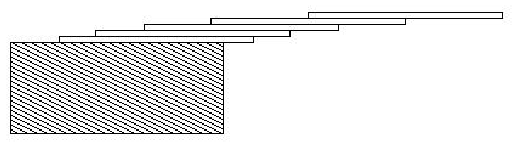# 一、题目

How far can you make a stack of cards overhang a table? If you have one card, you can create a maximum overhang of half a card length. (We're assuming that the cards must be perpendicular to the table.) With two cards you can make the top card overhang the bottom one by half a card length, and the bottom one overhang the table by a third of a card length, for a total maximum overhang of 1/2 + 1/3 = 5/6 card lengths. In general you can make n cards overhang by 1/2 + 1/3 + 1/4 + ... + 1/(n + 1) card lengths, where the top card overhangs the second by 1/2, the second overhangs tha third by 1/3, the third overhangs the fourth by 1/4, etc., and the bottom card overhangs the table by 1/(n + 1). This is illustrated in the figure below.The input consists of one or more test cases, followed by a line containing the number 0.00 that signals the end of the input. Each test case is a single line containing a positive floating-point number c whose value is at least 0.01 and at most 5.20; c will contain exactly three digits.

For each test case, output the minimum number of cards necessary to achieve an overhang of at least c card lengths. Use the exact output format shown in the examples.

Example input:

1.00
3.71
0.04
5.19
0.00

Example output:

3 card(s)
61 card(s)
1 card(s)
273 card(s)

# 二、AC代码

import java.util.Scanner;

/**
* @author ChangSheng
* @date 2020-03-28
*/
public class P0045_AC_HangOver {
public static void main(String[] args) {
Scanner sc = new Scanner(System.in);
while (sc.hasNext()) {
double sum = 0;
double temp = sc.nextDouble();
if (temp == 0) {
return;
}
for (int i = 0; i < 800; i++) {
sum = sum + 1.0 / (i + 2);
if (sum > temp) {
System.out.println((i + 1) + " card(s)");
break;
}
}
}
}
}